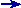OperatorsOperators are used as a means for object composition and embedding. Simple parsers may be composed to form composites through operator overloading, crafted to approximate the syntax of an Extended Backus-Normal Form (EBNF) variant. An expression such as:

``    a | b``

actually yields a new parser type which is a composite of its operands, a and b. Taking this example further, if a and b were of type chlit<>, the result would have the composite type:

``    alternative<chlit<>, chlit<> >``

In general, for any binary operator, it will take its two arguments, parser1 and parser2, and create a new composed parser of the form

``    op<parser1, parser2>``

where parser1 and parser2 can be arbitrarily complex parsers themselves, with the only limitations being what your compiler imposes.

### Set Operators

 Set operators ```a | b``` Union Match a or b. Also referred to as alternative ```a & b``` Intersection Match a and b ```a - b``` Difference Match a but not b. If both match and b's matched text is shorter than a's matched text, a successful match is made ```a ^ b``` XOR Match a or b, but not both

Short-circuiting

Alternative operands are tried one by one on a first come first served basis starting from the leftmost operand. After a successfully matched alternative is found, the parser concludes its search, essentially short-circuiting the search for other potentially viable candidates. This short-circuiting implicitly gives the highest priority to the leftmost alternative.

Short-circuiting is done in the same manner as C or C++'s logical expressions; e.g. if (x < 3 || y < 2) where, if x evaluates to be less than 3, the y < 2 test is not done at all. In addition to providing an implicit priority rule for alternatives which is necessary, given the non-deterministic nature of the Spirit parser compiler, short-circuiting improves the execution time. If the order of your alternatives is logically irrelevant, strive to put the (expected) most common choice first for maximum efficiency.Intersections Some researchers assert that the intersections (e.g. a & b) let us define context sensitive languages ("XBNF" [citing Leu-Weiner, 1973]). "The theory of defining a language as the intersection of a finite number of context free languages was developed by Leu and Weiner in 1973".~ Operator The complement operator ~ was originally put into consideration. Further understanding of its value and meaning leads us to uncertainty. The basic problem stems from the fact that ~a will yield U-a, where U is the universal set of all strings. However, where it makes sense, some parsers can be complemented (see the primitive character parsers for examples).

### Sequencing Operators

 Sequencing operators ```a >> b``` Sequence Match a and b in sequence ```a && b``` Sequential-and Sequential-and. Same as above, match a and b in sequence ```a || b``` Sequential-or Match a or b in sequence

The sequencing operator >> can alternatively be thought of as the sequential-and operator. The expression a && b reads as match a and b in sequence. Continuing this logic, we can also have a sequential-or operator where the expression a || b reads as match a or b and in sequence. That is, if both a and b match, it must be in sequence; this is equivalent to a >> !b | b.

### Optional and Loops

 Optional and Loops `*a` Kleene star Match a zero (0) or more times `+a` Positive Match a one (1) or more times `!a` Optional Match a zero (0) or one (1) time ```a % b``` List Match a list of one or more repetitions of a separated by occurrences of b. This is the same as a >> *(b >> a). Note that a must not also match bIf we look more closely, take note that we generalized the optional expression of the form !a in the same category as loops. This is logical, considering that the optional matches the expression following it zero (0) or one (1) time.

Primitive type operands

For binary operators, one of the operands but not both may be a char, wchar_t, char const* or wchar_t const*. Where P is a parser object, here are some examples:

``````    P | 'x'
P - L"Hello World"
'x' >> P
"bebop" >> P``````

It is important to emphasize that C++ mandates that operators may only be overloaded if at least one argument is a user-defined type. Typically, in an expression involving multiple operators, explicitly typing the leftmost operand as a parser is enough to cause propagation to all the rest of the operands to its right to be regarded as parsers. Examples:

``````    r = 'a' | 'b' | 'c' | 'd';          // ill formed
r = ch_p('a')  | 'b' | 'c' | 'd';   // OK``````

The second case is parsed as follows:

``````    r(((chlit<char> | char) | char) | char)

a(chlit<char> | char)
r(((a) | char) | char)

b(a | char)
r(((b)) | char)

c(b | char)
r(((c)))``````

Operator precedence and grouping

Since we are defining our meta-language in C++, we follow C/C++'s operator precedence rules. Grouping expressions inside the parentheses override this (e.g., *(a | b) reads: match a or b zero (0) or more times).